+650
6.5 to 1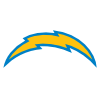Justin Herbert
9.0% implied probability

+800
8 to 1Tom Brady
7.5% implied probability

+800
8 to 1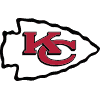Patrick Mahomes
7.5% implied probability

+1000
10 to 1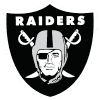Derek Carr
6.1% implied probability

+1000
10 to 1Matthew Stafford
6.1% implied probability

+1200
12 to 1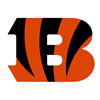Joe Burrow
5.2% implied probability

+1200
12 to 1Josh Allen
5.2% implied probability

+1400
14 to 1Dak Prescott
4.5% implied probability

+1600
16 to 1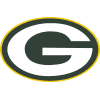Aaron Rodgers
4.0% implied probability

+1800
18 to 1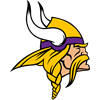Kirk Cousins
3.5% implied probability

+2000
20 to 1Russell Wilson
3.2% implied probability

+2500
25 to 1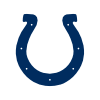Matt Ryan
2.6% implied probability

+2800
28 to 1Kyler Murray
2.3% implied probability

+3000
30 to 1Mac Jones
2.2% implied probability

+3000
30 to 1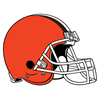Deshaun Watson
2.2% implied probability

+3000
30 to 1Jameis Winston
2.2% implied probability

+3000
30 to 1Trevor Lawrence
2.2% implied probability

+3000
30 to 1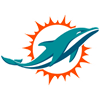Tua Tagovailoa
2.2% implied probability

+4000
40 to 1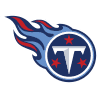Ryan Tannehill
1.6% implied probability

+4000
40 to 1Jalen Hurts
1.6% implied probability

+4000
40 to 1Carson Wentz
1.6% implied probability

+5000
50 to 1Lamar Jackson
1.3% implied probability

+5000
50 to 1Trey Lance
1.3% implied probability

+5000
50 to 1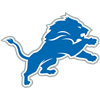Jared Goff
1.3% implied probability

+6000
60 to 1Zach Wilson
1.1% implied probability

+6000
60 to 1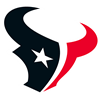Davis Mills
1.1% implied probability

+6000
60 to 1Mitch Trubisky
1.1% implied probability

+6000
60 to 1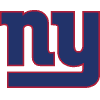Daniel Jones
1.1% implied probability

+6500
65 to 1Baker Mayfield
1.0% implied probability

+7000
70 to 1Jimmy Garoppolo
0.9% implied probability

+10000
100 to 1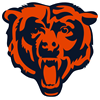Justin Fields
0.7% implied probability

+10000
100 to 1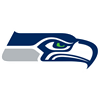Drew Lock
0.7% implied probability

+13000
130 to 1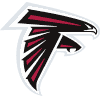Marcus Mariota
0.5% implied probability

+15000
150 to 1Andy Dalton
0.4% implied probability

+15000
150 to 1Teddy Bridgewater
0.4% implied probability

+15000
150 to 1Jordan Love
0.4% implied probability

+15000
150 to 1Sam Darnold
0.4% implied probability

+20000
200 to 1Taylor Heinicke
0.3% implied probability

+20000
200 to 1Taysom Hill
0.3% implied probability

+20000
200 to 1Gardner Minshew
0.3% implied probability

+20000
200 to 1Tyler Huntley
0.3% implied probability

+20000
200 to 1Tyrod Taylor
0.3% implied probability

+20000
200 to 1Mason Rudolph
0.3% implied probability

+20000
200 to 1Sam Howell
0.3% implied probability

+20000
200 to 1Kenny Pickett
0.3% implied probability

+20000
200 to 1Malik Willis
0.3% implied probability

+25000
250 to 1Cam Newton
0.3% implied probability

+25000
250 to 1Matt Corral
0.3% implied probability